Courses

# Chapter 8 Properties of Pure Substance - Thermodynamics, Mechanical Engineering Mechanical Engineering Notes | EduRev

## Mechanical Engineering : Chapter 8 Properties of Pure Substance - Thermodynamics, Mechanical Engineering Mechanical Engineering Notes | EduRev

The document Chapter 8 Properties of Pure Substance - Thermodynamics, Mechanical Engineering Mechanical Engineering Notes | EduRev is a part of the Mechanical Engineering Course Mechanical Engineering SSC JE (Technical).
All you need of Mechanical Engineering at this link: Mechanical Engineering

PROPERTIES OF PURE SUBSTANCE

• A pure susbtance is a substance of homogeneous chemical composition throughout its mass. It is one component system. It may exist in one or more phases.
•  A saturation state is a state from where a change of phase may occur without a changes of pressure or temperature.
•  Critical Point:
•  Critical Temperature : At critical temperature a liquid completely changes to vapour and viceversa. Also above critical temperature a vapour cannot be liquified by any amount of pressure.
•  Critical Pressure : At critical temperature the minimum pressure required to transform a vapour to liquid is called critical pressure.
•  Transformation of solid to vapour directly is called sublimation.
•  Transformation of vapour to solid directly is called ablimation.
•  Triple point is the fixed point (fixed temperature and pressure) at which solid, liquid and vapour phase co-exist in equilibrium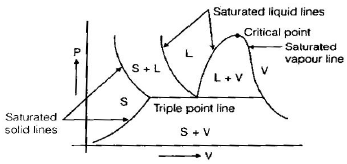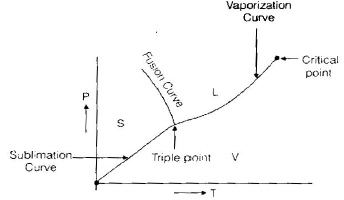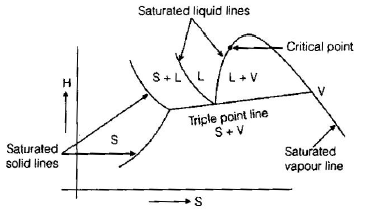• Following is the decreasing arrangement of substances triple point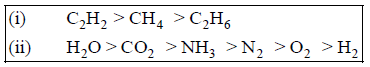• For water
•  Critical pressure (pc) = 221.2 bar
•  Critical temperature (tc) = 374.15ºC
•  Critical volume (vc) = 0.00317 m3/kg
•  Triple point (P) = 4.58 mm Hg
•  Triple point (T) = 273.16 K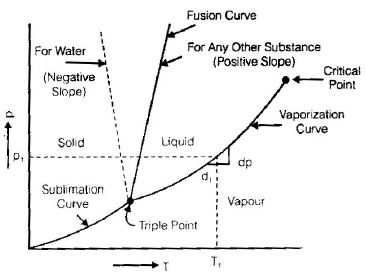• The slope of vaporization and sublimation curve for all substance are positive. The slope of the fusion curve for most substances is positive but for water, it is negative.
•  Water expands upon freezing while volume of other substances decreases upon freezing.
•  Mollier diagram (or h-s diagram)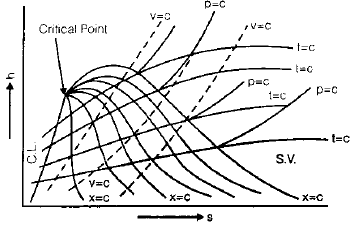• The slope of constant pressure lines is given as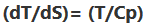• Dryness fraction : It is indicates the mass fraction of vapour in a liquid.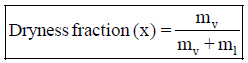mv = mass of vapour
ml = mass of liquid.

•  For saturated liquid, x = 0
•  For saturated vapour, x = 1
•  Dryness quality lines originate from critical line in various diagrams (P–V, h–s diagram etc.).

•  Various properties of pure substance based on dryness fraction.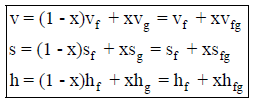• For given pressure and temperature

v, vf, vg = specific volume of moist vapour, liquid, vapour
vfg = vg – vf
h, hf, hg = specific enthalpy of moist vapour,liquid, vapour
hfg = hg – hf
s, sf, s= specific entropy of moist vapour, liquid, vapour
sfg = sg – sf

•  At triple point
 internal energy = 0entropy = 0enthalpy > 0
• A gas or a pure susbtance require two known properties (P, V, T, H etc.) to describe it completely i.e. it have two degrees of freedom.
•  A liquid and vapour in equilibrium state (saturated state) has one degree of freedom.
•  A liquid, vapour and solid in equillibrium (triple point) has zero degree of freedom.
•  The difference between the temperature of the superheated vapour and that of saturated vapour at the same pressure is called degree of superheat.
•  Throtting caloriemter
•  It is used to measure dryness fraction of pure substance.
•  Throttling is an irreversible and adiabatic but not isentropic.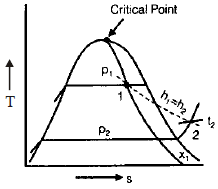• It is very difficult to measure quality of two phase system (liquid+vapour) so it is throttled to bring it to single phase that is vapour phase and then the measurment of pressure, temperature and other properties of two phase system can be done.
Offer running on EduRev: Apply code STAYHOME200 to get INR 200 off on our premium plan EduRev Infinity!

83 docs|63 tests

,

,

,

,

,

,

,

,

,

,

,

,

,

,

,

,

,

,

,

,

,

,

,

,

;